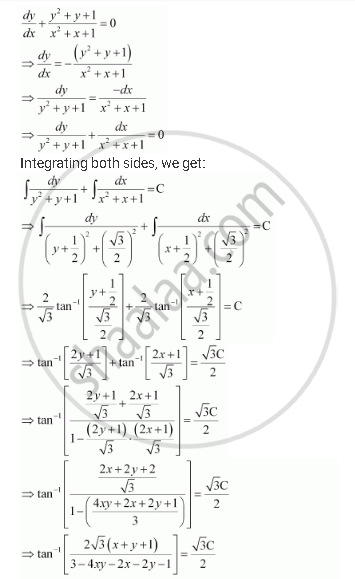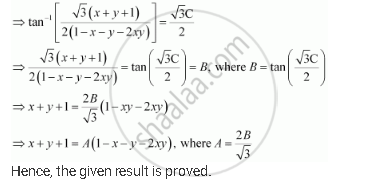Share

# Show that the General Solution of the Differential Equation Dy/Dx + (Y^2 + Y +1)/(X^2 + X + 1) = 0 Is Given by (X + Y + 1) = A (1 – X – Y – 2xy), Where A Is Parameter - Mathematics

#### Question

Show that the general solution of the differential equation  dy/dx + (y^2 + y +1)/(x^2 + x + 1) = 0 is given by (x + + 1) = (1 – – y – 2xy), where is parameter

#### SolutionIs there an error in this question or solution?
Solution Show that the General Solution of the Differential Equation Dy/Dx + (Y^2 + Y +1)/(X^2 + X + 1) = 0 Is Given by (X + Y + 1) = A (1 – X – Y – 2xy), Where A Is Parameter Concept: General and Particular Solutions of a Differential Equation.